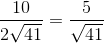## Example Questions

### Example Question #91 : Trigonometry

In the triangle below,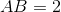units and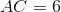units. What is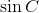?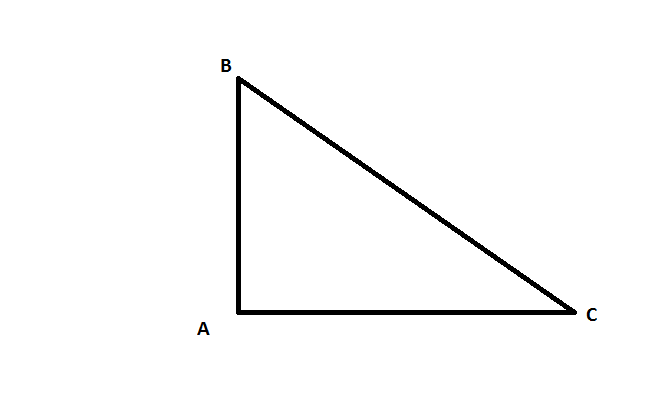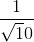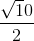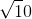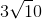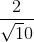Explanation:

Because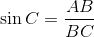, we need to first find the length of BC.

Using the Pythagorean theorem,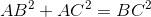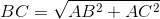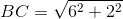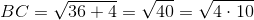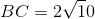.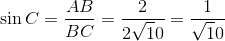### Example Question #1 : How To Find The Sine Of A Missing Side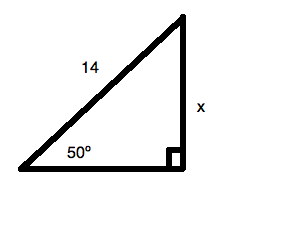What is the value ofin the triangle above? Round to the nearest hundredth.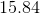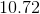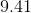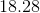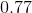Explanation:

We know that the sine of an angle is: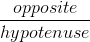Therefore, we can write for this question: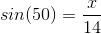This allows us to solve foreasily: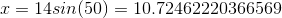Rounding, this gives us.

### Example Question #93 : Trigonometry

A right triangle has leg lengthsand. What is the sine of the angle opposite from the side of length?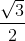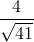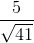Explanation:

Using SOHCAHTOA, the sine of an angle is simply the length of the side opposite to it over the hypotenuse; however, we do not have the length of the hypotenuse yet.

Using the Pythagorean Theorem, we can solve for it: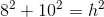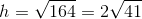So, the sine of this angle is: